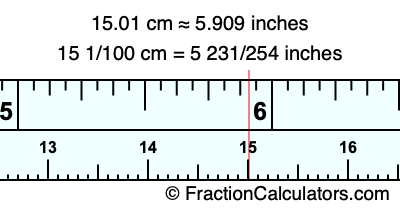15.01 cm in inchesHere is how to convert 15.01 cm to inches. We will give you the fractional answer, the decimal answer, and illustrate the answer on our tape measure.

One centimeter is equal to 50/127 inches, and 15.01 as a fraction is 15 1/100. Therefore, to get the fractional answer to 15.01 cm in inches, we multiply 15 1/100 by 50/127 and simplify if necessary.

cm × 50/127 = inches
15 1/100 × 50/127 = inches
15 1/100 × 50/127 = 5 231/254
15 1/100 cm = 5 231/254 inches
15.01 cm = 5 231/254 inches

As promised above, we also have the answer to 15.01 cm in inches in decimal form. Although the fractional answer above is exact, the decimal answer may be rounded if necessary:

5 231/254 ≈ 5.90944881889764
5 231/254 ≈ 5.909
15.01 cm ≈ 5.909 inches

Our image below shows 15.01 cm on a tape measure. The top row of the tape measure is inches, and the bottom row is centimeters.As you can see, we drew a red line where 15.01 cm and 5.909 inches meet on the measuring tape.

Centimeters to Inches Calculator
Here you can convert another length in centimeters to inches.

Convert  cm to inches.

What is 15.02 cm in inches?
Here is another inches to centimeters calculation we did for you.